Courses

# NCERT Solutions - Force and Laws of Motion, Science, Class 9 Class 9 Notes | EduRev

## Class 9 : NCERT Solutions - Force and Laws of Motion, Science, Class 9 Class 9 Notes | EduRev

The document NCERT Solutions - Force and Laws of Motion, Science, Class 9 Class 9 Notes | EduRev is a part of the Class 9 Course Class 9 Science by VP Classes.
All you need of Class 9 at this link: Class 9

Q1. Which of the following has more inertia:
(a) a rubber ball and a stone of the same size?
(b) a bicycle and a train?
(c) a five-rupees coin and a one-rupee coin?
Ans
. (a) A stone of the same size
(b) a train
(c) a five-rupees coin As the mass of an object is a measure of its inertia, objects with more mass have more inertia.

Q2. In the following example, try to identify the number of times the velocity of the ball changes. “A football player kicks a football to another player of his team who kicks the football towards the goal. The goalkeeper of the opposite team collects the football and kicks it towards a player of his own team”.
Also identify the agent supplying the force in each case.
Ans.

 Agent supplying the force Change in velocity of ball 1. First player kicks a football. → Velocity from ‘0’ changes to ‘u’ 2. Second player kicks the football towards the goal. → Velocity changes again 3. The goalkeeper collects the football. → Velocity becomes 0 4. Goalkeeper kicks it towards a player of his team. → Change in velocity takes place

The velocity of football changed four times.

Q3. Explain why some of the leaves may get detached from a tree if we vigorously shake its branch.
Ans.
When the tree’s branch is shaken vigorously the branch attain motion but the leaves stay at rest. Due to the inertia of rest, the leaves tend to remain in its position and hence detaches from the tree to fall down.

Q4. Why do you fall in the forward direction when a moving bus apply brakes to stop and fall backwards when it accelerates from rest?
Ans.
When a moving bus applies brakes to stop: When the bus is moving, our body is also in motion, but due to sudden brakes, the lower part of our body comes to rest as soon as the bus stops. But the upper part of our body continues to be in motion and hence we fall in forward direction due to inertia of motion.
When the bus accelerates from rest we fall backwards: When the bus is stationary our body is at rest but when the bus accelerates, the lower part of our body being in contact with the floor of the bus comes in motion, but the upper part of our body remains at rest due to inertia of rest. Hence we fall in backward direction.

Q5. If action is always equal to the reaction, explain how a horse can pull a cart?
Ans.
The third law of motion states that action is always equal to the reaction but they act on two different bodies.
In this case the horse exerts a force on the ground with its feet while walking, the ground exerts an equal and opposite force on the feet of the horse, which enables the horse to move forward and the cart is pulled by the horse.

Q6. Explain, why is it difficult for a fireman to hold a hose, which ejects a large amount of water at a high velocity.
Ans.
The water that is ejected out from the hose in the forward direction comes out with a large momentum and equal amount of momentum is developed in the hose in the opposite direction and hence the hose is pushed backward. It becomes difficult for a fireman to hold a hose which experiences this large momentum.

Q7. From a rifle of mass 4 kg, a bullet of mass 50 g is fired with an initial velocity of 35 m/s. Calculate the initial recoil velocity of the rifle.
Ans.
(m1) Mass of rifle = 4 kg (m2)
Mass of bullet = 50 g = 0.05 kg (v2)
Velocity of bullet = 35 m/s (v1)
Recoil velocity of rifle = ?
According to the law of conservation of momentum Momentum of rifle = momentum of bullet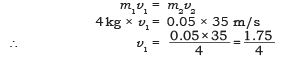v1 = 0.4375 m/s ∴ Recoil velocity of rifle = 0.4375 m/s

Q8. Two objects of masses 100 g and 200 g are moving along the same line and direction with velocities of 2m/s and 1m/s respectively.
They collide and after the collision the first object moves at a velocity of 1.67 m/s. Determine the velocity of the second object.
Ans.
m1 = 100 g = 0.1 kg m2 = 200 g = 0.2 kg u1 = 2 m/s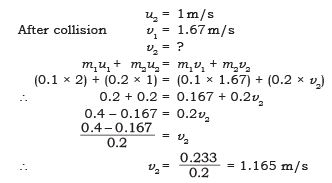∴ The velocity of the second object is 1.165 m/s.

NCERT Textual Questions Solved.

Q1. An object experiences a net zero external unbalanced force. Is it possible for the object to be travelling with a non-zero velocity? If yes, state the conditions that must be placed on the magnitude and direction of the velocity. If no, provide a reason.
Ans.
When an object experiences a net zero external unbalanced force, in accordance with second law of motion its acceleration is zero. If the object was initially in a state of motion, then in accordance with the first law of motion, the object will continue to move in same direction with same speed. It means that the object may be travelling with a non-zero velocity but the magnitude as well as direction of velocity must remain unchanged or constant throughout.

Q2. When a carpet is beaten with a stick, dust comes out of it. Explain.
Ans.
The carpet with dust is in state of rest. When it is beaten with a stick the carpet is set in motion, but the dust particles remain at rest. Due to inertia of rest the dust particles retain their position of rest and falls down due to gravity.

Q3. Why is it advised to tie any luggage kept on the roof of a bus with a rope?
Ans.
In moving vehicle like bus, the motion is not uniform, the speed of vehicle varies and it may apply brake suddenly or takes sudden turn. The luggage will resist any change in its state of rest or motion, due to inertia and this luggage has the tendency to fall sideways, forward or backward.
To avoid the fall of the luggage, it is tied with the rope.

Q4. A batsman hits a cricket ball which then rolls on a level ground. After covering a short distance, the ball comes to rest. The ball slows to a stop because
(a) the batsman did not hit the ball hard enough.
(b) velocity is proportional to the force exerted on the ball.
(c) there is a force on the ball opposing the motion.
(d) there is no unbalanced force on the ball, so the ball would want to come to rest.
Ans.
(c) there is a force on the ball opposing the motion.

Q5. A truck starts from rest and rolls down a hill with a constant acceleration. It travels a distance of 400 m in 20 s. Find its acceleration. Find the force acting on it if its mass is 7 tonnes (Hint : 1 tonne = 1000kg).
Ans.
u = 0 m/s m = 7 tonnes s = 400 m = 7 × 1000 kg = 7000 kg t = 20 s a = ?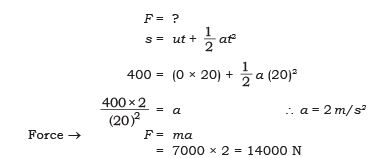Q6. A stone of 1kg is thrown with a velocity of 20 ms–1 across the frozen surface of a lake and comes to rest after travelling a distance of 50 m. What is the force of friction between the stone and the ice?
Ans.

m = 1 kg

u= 20m/s

s= 50m

v= 0

F = ?

a = ?

v2 - u2 = 2sa

(o)2 - (20)2 = 2a (50)

∴ -400 = 100a

∴  a = -400/100 = -4m/s2

Force of friction, F = m x a

=1kg x -4m/s2

= - 4 N

Q7. A 8000 kg engine pulls a train of 5 wagons, each of 2000 kg, along a horizontal track. If the engine exerts a force of 40000 N and the track offers a friction force of 5000 N, then calculate:
(a) the net accelerating force; and
(b) the acceleration of the train.
Ans.
(a) The net accelerating force = Force exerted by the engine – friction force
= 40000 N – 5000 N
= 35000 N
(b) The acceleration of the train (a) = ?
F = 35000 N
Mass of train = Mass of 5 wagons + Engine = 5 × 2000 + 8000
= 10000 kg + 8000 kg = 18000 kg
∴ F = ma 35000 = 18000 × a
∴ a = (35000/18000)= 1.94 m/s2

Q8. An automobile vehicle has a mass of 1500 kg. What must be the force between the vehicle and road if the vehicle is to be stopped with a negative acceleration of 1.7 ms–2?
Ans.
mass = 1500 kg
a = –1.7 m/s2
F = ?
F = m × a = 1500 × (– 1.7)
= – 2550 N
The force between the vehicle and road is – 2550 N.

Q9. What is the momentum of an object of mass m, moving with a velocity v?
(a) (mv)2
(b) mv2
(c) 1/2 mv2
(d) mv
Ans. (d) mv

Q10. Using a horizontal force of 200 N, we intend to move a wooden cabinet across a floor at a constant velocity. What is the friction force that will be exerted on the cabinet?
Ans.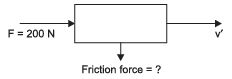As the wooden cabinet moves across the floor at a constant velocity and the  force applied is 200 N. Hence the frictional force that will be exerted on the cabinet will be less than 200 N.

Q11. Two objects each of mass 1.5 kg, are moving in the same straight line but in opposite directions. The velocity of each object is 2.5 ms–1 before the collision during which they stick together. What will be the velocity of the combined object after collision?
Ans.
Mass of the objects m= m2 = 1.5 kg
Velocity of first object u1 = 2.5 m/s
Velocity of second object u2 = – 2.5 m/s
Momentum before collision = m1u1 + m2u2 = (1.5 × 2.5) + (1.5 × –2.5) = 0
Mass after collision = m1 + m2 = 1.5 + 1.5 = 3.0 kg
After collision v = ?
According to law of conservation of momentum
Momentum before collision = Momentum after collision
0 = 3 × v
∴ v = 0 m/s

Q12. According to the third law of motion when we push on an object, the object pushes back on us with an equal and opposite force. If the object is a massive truck parked along the roadside, it will probably not move. A student justifies this by answering that the two opposite and equal forces cancel each other. Comment on this logic and explain why the truck does not move.
Ans.
The mass of truck is too large and hence its inertia is too high. The small force exerted on the truck cannot move it and the truck remains at rest. For the truck to attain motion, an external large amount of unbalanced force need to be exerted on it.

Q13. A hockey ball of mass 200 g travelling at 10 ms–1 is struck by a hockey stick so as to return it along its original path with a velocity at 5 ms–1. Calculate the magnitude of change of momentum occurred in the motion of the hockey ball by the force applied by the hockey stick.
Ans.
Mass of ball m = 200 g = 0.2 kg Initial speed of ball u = 10 m/s
Final speed of ball v = – 5 m/s
Initial momentum of the ball = mu = 0.2 kg × 10 m/s = 2 kg m/s
Final momentum of the ball = mv = 0.2 kg × (– 5 m/s) = – 1 kg m/s
Hence, change in momentum = Difference in the momentum = – 1 – 2 = – 3 kg m/s

Q14. A bullet of mass 10 g travelling horizontally with a velocity of 150 ms–1 strikes a stationary wooden block and comes to rest in 0.03 s. Calculate the distance of penetration of the bullet into the block. Also calculate the magnitude of the force exerted by the wooden block on the bullet.
Ans
. m = 10 g = (10/1000)= 0.01 kg
u = 150 m/s
v = 0 m/s
t = 0.03 s
v = u + at
0 = 150 + a (0.03)
a = (-150/0.03)= –5000m/s2 s = ?
F = ?
v2 – u2 = 2as
∴ (0)2 – (150)2 = 2 × 5000 × s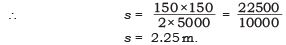∴    The penetration distance of the bullet in the wooden block = 2.25 m.
Magnitude of force F = ma = 0.01 × (– 5000)
F = – 50 N.

Q15. An object of mass 1 kg travelling in a straight line with a velocity of 10 ms–1 collides with, and sticks to, a stationary wooden block of mass 5kg. Then they both move off together in the same straight line. Calculate the total momentum just before the impact and just after the impact. Also, calculate the velocity of the combined object.
Ans.

m1 = 1 kg
v1 = 10 m/s
Mass of wooden block = 5 kg m2 = 5 kg + 1 kg (combined object) = 6 kg
Velocity of combined object = v2 = ? p1 and p2 = ?
Momentum before impact p1 = m1v1 = 1 × 10 = 10 kg m/s
∴ Momentum before impact = Momentum after impact m1v1 = m2v2
10 kg m/s = 6 v2
∴ (10/6)= v2
∴  v= 1.67 m/s

Q16. An object of mass 100 kg is accelerated uniformly from a velocity of 5 ms–1 to 8 ms–1 in 6 s. Calculate the initial and final momentum of the object. Also, find the magnitude of the force exerted on the object.
Ans.

m = 100 kg
u = 5 m/s
v = 8 m/s
t = 6 s
p1 = ?
p2 = ?

F = ?

∴ Initial momentum p1 = mu = 100 × 5 = 500 kg m/s

Final momentum p2 = mv = 100 × 8 = 800 kg m/s

Force exerted on the object F = ma = 100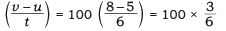F = 50 N

Q17. Akhtar, Kiran and Rahul were riding in a motorcar that was moving with a high velocity on an expressway when an insect hit the windshield and got stuck on the windscreen. Akhtar and Kiran started pondering over the situation. Kiran suggested that the insect suffered a greater change in momentum as compared to the change in momentum of the motorcar (because the change in the velocity of insect was much more than that of the motorcar). Akhtar said that since the motorcar was moving with a larger velocity, it exerted a larger force on the insect. And as a result the insect died. Rahul while putting an entirely new explanation said that both the motorcar and the insect experienced the same force and a change in their momentum. Comment on these suggestions.
Ans.
Rahul gave the correct reasoning and explanation that both the motorcar and the insect experienced the same force and a change in their momentum. As per the law of conservation of momentum

When two bodies collide: Initial momentum before collision = Final momentum after collision

m1u1 + m2u= m1v1 + m2v2

The equal force is exerted on both the bodies but, because the mass of insect is very small it will suffer greater change in velocity.

Q18. How much momentum will a dumb-bell of mass 10 kg transfer to the floor if it falls from a height of 80 cm? Take its downward acceleration to be 10 ms–2.
Ans.
Mass of dumb-bell = 10 kg
Height, h = 80 cm = 0.8 m
g = 10 m/s2
u = 0
v2 – u2 = 2gh
v2 – (0)= 2 × 10 × 0.8
v2 = 16
∴ v = 4 m/s
Momentum p = mv = 10 × 4 = 40 kg m/s

Offer running on EduRev: Apply code STAYHOME200 to get INR 200 off on our premium plan EduRev Infinity!

75 docs|14 tests

,

,

,

,

,

,

,

,

,

,

,

,

,

,

,

,

,

,

,

,

,

,

,

,

,

,

,

;